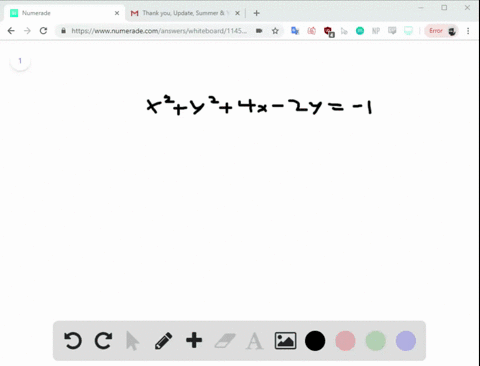Enroll in one of our FREE online STEM summer camps. Space is limited so join now!View Summer Courses### $$x^{2}+y^{2}+4 x-2 y=-1$$ The equation of a cir…

02:04Johns Hopkins University

Need more help? Fill out this quick form to get professional live tutoring.

Get live tutoring
Problem 23

As part of an experiment, a ball was dropped and
allowed to bounce repeatedly off the ground until it
came to rest. The graph above represents the
relationship between the time elapsed after the ball
was dropped and the height of the ball above the
ground. After it was dropped, how many times was
the ball at a height of 2 feet?
$$\begin{array}{l}{\text { A) One }} \\ {\text { B) Two }} \\ {\text { C) Three }} \\ {\text { D) Four }}\end{array}$$

Check back soon!
SAT
SAT Practice Test # 6

## Discussion

You must be signed in to discuss.

## Video Transcript

And so for this one, we want to know how many times after it was dropped was the ball at a height of two feet, so we won't know at a height of two feet. Well, two feet is here. We can draw this line all the way across and see how many times the ball across that two line point. It's kind of like if you were taking like, a little ribbon and you had it at two feet and you see how many times the ball crosses that as it goes up, down and up and down, up and down. Well, for here, across this once, twice, three times so you know it's gonna be C three times.